### Problem 1

IQIZ estimated its factory overhead for the next period at \$160,000. It is estimated that 40,000 units will be produced at a materials cost of \$200,000.

Production will require 40,000 man-hours at an estimated wage cost of \$80,000. The machines will run for approximately 25,000 hours.

Required: Calculate the factory overhead rate that may be used in applying FOH to production on each of the following bases:

1. Materials cost
2. Direct labor cost
3. Direct labor hours
4. Machine hours
5. Units of production
6. Prime cost

### Solution

1. Material Cost Basis
Formula:
= (Estimated factory overhead / Estimated material cost) x 100
= (\$160,000 / \$200,000) x 100
= 80%
2. Direct Labor Cost Basis
Formula:
= (Estimated FOH / Estimated DL cost) x 100
= (\$160,000 / \$80,000) x 100
= 200%
3. Direct Labor Hours Basis
Formula:
= (Estimated FOH / Estimated DL hours) x 100
= \$160,000 / 40,000 hrs.)
= \$4.00 per hour
4. Machine Hours Basis
Formula:
= Estimated FOH / Estimated machine hours
= \$160,000 / 25,000 hrs.
= \$6.40 per machine hour
5. Units of Production Cost
Formula:
= Estimated FOH / Estimated no. of units
= \$160,000 / 40,000 hours
= \$4.00 per unit
6. Prime Cost Basis
Formula:
= Estimated FOH / Estimated prime cost
= (\$160,000 / (\$200,000 + 80,000)) x 100
= 89%

## FOH Variances

### Problem 2

The factory overhead for the King Manufacturing Company is estimated as follows:

• Estimated direct labor hours = 20,000

Production for the month reached 75% of the budget. In addition, actual factory overhead totalled \$43,000.

Required: Calculate the following:

1. Applied factory overhead (i.e., overapplied or underapplied)
2. Spending and capacity variances

### Working

FOH Applied Rate
Formula:
= FOH applied for normal capacity / Normal capacity
= \$60,000 (15,000 + 45,000) / 20,000 hrs.
= \$3 per hour
Applied FOH for Actual Capacity or Capacity Attained
Formula:
= Actual capacity x FOH hrs. x \$3
= (20,000 x 75%) x \$3
= 15000 hrs. x \$3
= \$45,000
Budgeted Allowance
Formula:
= Fixed cost + Variable cost for actual capacity
= \$15,000 + 33,750*
= \$48,750
* Variable Cost for Actual Capacity
Formula:
= Actual capacity x Variable cost rate
= 15,000 x \$2.25*
= \$33,750
* Variable Cost Rate
Formula:
= Variable cost for normal volume / Normal volume
= \$45,000 / 20,000 hrs.
=\$2.25 per hour

### Solution

1. Overapplied or underapplied FOH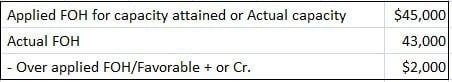2. Variances

Spending variance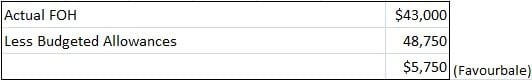Capacity variance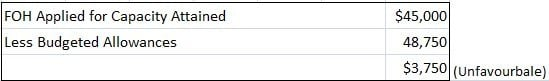Variance check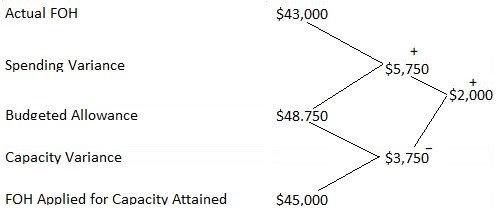## High-Low Point Method

### Problem 3

The burden rate of John & Co. is \$2.00 per hour. The budgeted overhead for 3,000 hours per month is \$8,000 and at 7,000 hours is \$12,000. Actual factory overhead for the month was \$9,000 and actual volume was 5,000 hours.

Required: Calculate the following:

1. Variable overhead in burden rate
3. Normal volume
6. Idle capacity variance
7. Spending variance

### Solution

 Activity Level Budgeted FOH (hrs.) (\$) 7,000 12,000 3,000 8,000 4,000 4,000

#### 1. Variable Cost Rate/V.C. in burden rate

Formula:
= Difference in burden FOH / Difference in activity level
= \$4,000 / 4,000 hrs.
= \$1 per hour

 Budgeted FOH for 7,000 hrs. \$12,000 Less VC for 7,000 hrs. (7,000 x 1) \$7,000 Fixed Cost \$5,000 OR Budgeted FOH for 3,000 hours \$8,000 Less VC for 3,000 hours (3,000 x 1) \$3,000 Fixed Cost \$5,000

#### 3. Normal Volume/Standard Activity Level

Formula:
= Fixed FOH Cost / Fixed FOH Cost Rate
= \$5,000 / \$1
= 5,000 hrs.

Formula:
= Actual capacity x FOH applied rate
= 5,000 x 2
= \$10,000

#### 5. Over- or Under-absorbed FOH

 Applied FOH for Capacity Attained \$10,000 Less Actual FOH \$9,000 Overapplied FOH \$1,000

#### 6. Capacity Variance

 FOH Applied for Capacity Attained \$10,000 Less Budgeted Allowance \$10,000 0

#### 7. Spending Variance

 Actual FOH \$9,000 Less Budgeted Allowance \$10,000 1,000 (Favourable)

Variance check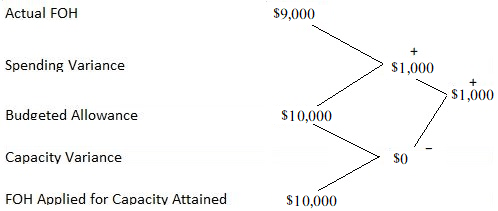Calculations

 Fixed FOH Rate Applied Burden Rate \$2.00 Less Variable Rate \$1.00 Fixed Burden Rate \$1.00

Budgeted allowance:
= Fixed cost + Variable cost for capacity attained
= 5,000 + (5,000 x 1)
= 5,000 + 5,000
= \$10,000

### Why does the high-low method work best with variable overhead?

High low works best with voh because it is easy to precisely determine the difference in activity level between two months (i.E., Number of hours worked).

### When do you use a predetermined overhead rate instead of allocation bases to assign factory overhead costs to production?

Using a predetermined overhead rate is better when assigning factory overheads to production in an environment where the activity levels for various products remains relatively constant even in periods of high and low sales.

### What does it mean when a company uses a variable costing system?

A variable costing system allows companies to understand costs based on how much they are affected by sales volume. In a variable costing system, overhead costs are typically charged to expense in the period when they are incurred rather than being spread out across all units produced over a specific time frame.

### How is the activity level used when assigning factory overhead?

Activity level is a predefined characteristic that can be applied in two different ways: an activity index or a level of capacity. These are used when allocating fixed overhead to multiple products based on the production volume associated with each unit. For example, an activity index can be calculated by dividing costs by units produced, while capacity is defined as hours worked over some predetermined time period.

### What are examples of an allocation base?

Examples of allocation bases include machine hours and direct labor hours.

True is a Certified Educator in Personal Finance (CEPF®), a member of the Society for Advancing Business Editing and Writing, contributes to his financial education site, Finance Strategists, and has spoken to various financial communities such as the CFA Institute, as well as university students like his Alma mater, Biola University, where he received a bachelor of science in business and data analytics.

To learn more about True, visit his personal website, view his author profile on Amazon, his interview on CBS, or check out his speaker profile on the CFA Institute website.